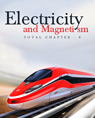Click to Chat

1800-1023-196

+91-120-4616500

CART 0

• 0

MY CART (5)

Use Coupon: CART20 and get 20% off on all online Study Material

ITEM
DETAILS
MRP
DISCOUNT
FINAL PRICE
Total Price: Rs.

There are no items in this cart.
Continue Shopping
`        A current of 1.0 A exists in a copper wire of cross-section 1.0 mm^2. Assuming one free electron per atom calculate the drift speed of the free electrons in the wire. The density of copper is 9000 kg/m^3.`
5 years ago

```							Sol. i = 1 A, A = 1 mm2 = 1 * 10^–6 m^2
F’ cu = 9000 kg/m^3
Molecular mass has N base 0 atoms
= m Kg has (N base 0/M * m) atoms = N base 0AI9000/36.5 * 10^-3
No.of atoms = No.of electrons
n = No.of electrons/Unit volume = N base 0Af/mAI = N base 0f/M
= 6 * 10^23 * 9000/63.5 * 10^-3
i = V base d n A e.
⇒ V base d = i/nAe = 1/6 * 10^23 *9000/63.3 * 10^-3 *10^-6 *1.6 * 10^-19
= 63.5 * 10^-3/6 * 10^23 *9000 * 10^-6 * 1.6 * 10^-19 = 63.5 * 10^-3/6 * 9 * 1.6 * 10^26 *10^-19 * 10^-6
= 63.5 * 10^10-3/6 * 9 * 1.6 * 10 = 63.5 * 10^-3/6 * 9 *16

```
5 years ago
```								Density of Cu=9×103kg/m3 (mass of 1 m3 of Cu) 6.0×1023 atoms has a mass = 63×103kg \ Number of electrons per m3are               =6.0×102363×10-3×9×103=8.5×10​28​​  Now drift velocity =vd=ineA =1.18.5×1028×1.6×10−19×π×(0.5×10−3)2 =0.1×10−3m/sec
```
one year ago
Think You Can Provide A Better Answer ?

## Other Related Questions on Electromagnetic Induction

View all Questions »### Course Features

• 731 Video Lectures
• Revision Notes
• Previous Year Papers
• Mind Map
• Study Planner
• NCERT Solutions
• Discussion Forum
• Test paper with Video Solution### Course Features

• 57 Video Lectures
• Revision Notes
• Test paper with Video Solution
• Mind Map
• Study Planner
• NCERT Solutions
• Discussion Forum
• Previous Year Exam Questions